Custom math worksheets at your fingertips# Our Top Ten lists

Just in case you are curious, to give you some inspiration, or just for browsing: Here are our Top Ten lists. These lists are created daily from current actual activity of our users.

Look at:

Join the crowd, register here.

## Top Ton most popular math problems

These are the problems that are used most to build customized worksheets.

Click the Quickname to go to the detail view page for the problem. You can download sample worksheets and solution sheets for this problem there, too. If you remember the problem's Quickname, you can add it to your own worksheet quickly: in the bottom right corner of the worksheet editor, there is a form field for it.

Place
Problem's name
Description
Quickname
Example
1.
Multiply fractions
Multiply two fractions.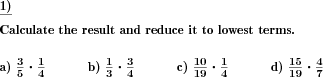2.
Divide two fractions
Division of two fractions.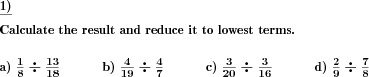3.
Name the 2d geometric shape
Name the two dimensional flat geometric shape shown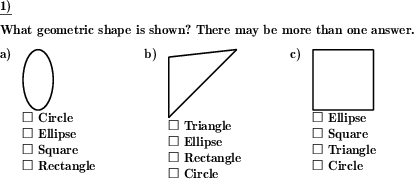4.
Labyrinth
Find your way through the labyrinth.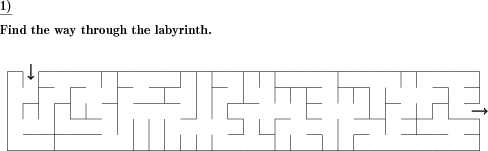5.
Rule of three word problem
Word problems that can be solved by applying the rule of three.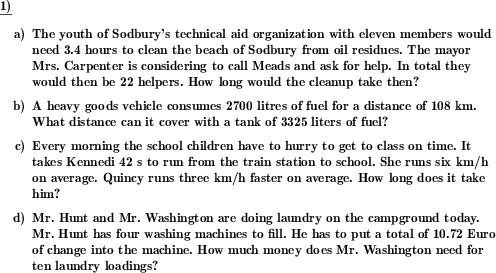6.
Fractions multiplication by whole numbers fill blanks
What whole number has be fraction been multiplied with?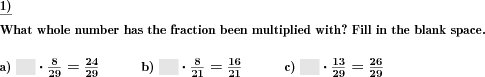7.
Triangle label parts
A fully or partly unlabeled triangle is given. The vertices, sides or angles have to be labeled correctly.8.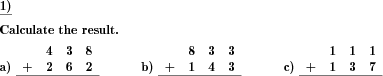9.
Name the 3d geometric shape
An oblique sketch of a geometric shape is shown. Name the shape.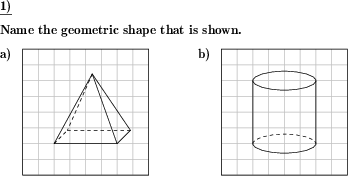10.
Clock faces, match digital and analog
Match pairs of analog and digital clock faces showing the same time.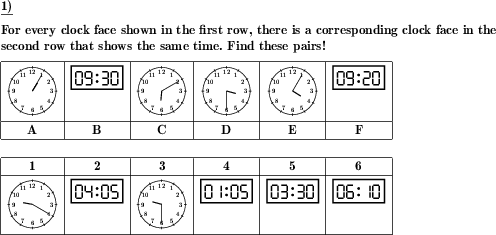## The top ten most frequently generated problems

Here at dw-math, math problems are generated automatically according to your needs. This is why all problems look different. These are the problems that our users have been re-generating the most during the last 60 days. This means that there are problems have been added to a worksheet or have been refreshed on existing worksheets frequently..

Click the Quickname to go to the detail view page for the problem. You can download sample worksheets and solution sheets for this problem there, too. If you remember the problem's Quickname, you can add it to your own worksheet quickly: in the bottom right corner of the worksheet editor, there is a form field for it.

Place
Problem's name
Description
Quickname
Example
1.
Multiply fractions
Multiply two fractions.2.
Divide two fractions
Division of two fractions.3.
Name the 2d geometric shape
Name the two dimensional flat geometric shape shown4.
Labyrinth
Find your way through the labyrinth.
13525.
Rule of three word problem
Word problems that can be solved by applying the rule of three.
38946.
Fractions multiplication by whole numbers fill blanks
What whole number has be fraction been multiplied with?
25017.
Triangle label parts
A fully or partly unlabeled triangle is given. The vertices, sides or angles have to be labeled correctly.
32338.
32909.
Name the 3d geometric shape
An oblique sketch of a geometric shape is shown. Name the shape.
597810.
Clock faces, match digital and analog
Match pairs of analog and digital clock faces showing the same time.
6912These informational pages with samples describe math problems that can be combined on custom math worksheets with solutions for home and K-12 school use.